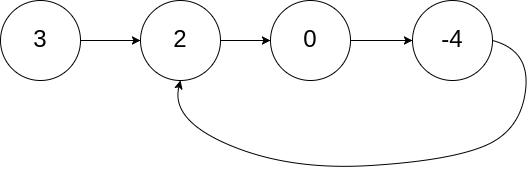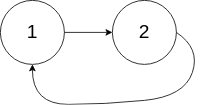For beginners, time is a bit rushed, many solutions are not analyzed in detail, and may be revised in the future, forgive me.
Datawhale learning document:
https://github.com/datawhalechina/team-learning-program/tree/master/LeetCodeTencent

136 Number that appears only once

Source: LeetCode

Given an array of non-empty integers, except for an element that appears only once, every other element appears twice. Find the element that appears only once.
Explanation:
Your algorithm should have linear time complexity. Can you do this without using extra space?

Example:

Input: [2,2,1]
Output: 1
Input: [4,1,2,1,2]
Output: 4

Ideas

Official problem solution: If there is no time complexity and space complexity limit, there are many solutions to this problem, the possible solutions are as follows.

• Use collections to store numbers. Traverse each number in the array. If there is no such number in the set, add the number to the set. If the number already exists in the set, delete the number from the set. The last remaining number is the number that appears only once .
• Use a hash table to store each number and the number of times that number appears. Traverse the array to get the number of occurrences of each number, update the hash table, and finally traverse the hash table to get the number that appears only once.
• Use a collection to store all the numbers that appear in the array, and calculate the sum of the elements in the array. Since the set guarantees that there is no repetition of elements, it is calculated twice the sum of all elements in the set, that is, the sum of the elements when each element appears twice. Since only one element in the array appears once, and the remaining elements appear twice, the sum of the elements in the array is subtracted from twice the sum of the elements in the set. The remaining number is the number that appears only once in the array.

Need to use additional O(n) space, in order to meet the linear time complexity, the official solution method is bit operation. The nature of XOR:

• Any number and 0 are XORed, the result is still the original number, that is, a⊕0=a
• XOR any number with itself, the result is 0, that is, a⊕a=0
• The exclusive OR operation satisfies the commutative and associative laws, that is,
a⊕b⊕a=b⊕a⊕a=b⊕(a⊕a)=b

Perform exclusive OR operation on all elements in the array. Commutative and associative laws allow us to move the number position at will without changing the result of the exclusive OR operation.
a1⊕a2⊕a2⊕a3⊕b⊕a3⊕a1 =(a1⊕a1)⊕(a2⊕a2)⊕(a3⊕a3)⊕b
= b
Finally, for any number that appears twice (or even number of times) Both can be converted into a⊕a=0, and the number that appears once (or an odd number of times, eliminated by 2n+1) is retained by b⊕0=b.
This question only appears once or twice, so the result of the exclusive OR operation of all elements in the array is the number that appears only once in the array.

Python implementation

(A line of code for the official solution)
The XOR operator is ^

class Solution:
def singleNumber(self, nums: List[int]) -> int:
return reduce(lambda x, y: x ^ y, nums)

Source: LeetCode

Given a linked list, determine whether there is a ring in the linked list.
If there is a node in the linked list that can be reached again by continuously tracking the next pointer, then there is a ring in the linked list. In order to represent the rings in a given linked list, we use the integer pos to indicate the position where the end of the linked list is connected to the linked list (the index starts from 0). If pos is -1, there are no rings in the linked list. **Note: pos is not passed as a parameter, ** is only to identify the actual situation of the linked list.
If there is a ring in the linked list, return true. Otherwise, it returns false.

Can you solve this problem with O(1) (ie, constant) memory?

Example:

Input: head = [3,2,0,-4], pos = 1
Output: true
Explanation: There is a ring in the linked list, and its tail is connected to the second node.Input: head = [1,2], pos = 0
Output: true
Explanation: There is a ring in the linked list, and its tail is connected to the first node.Input: head = , pos = -1
Output: false
Explanation: There is no ring in the linked list.

prompt:

The number of nodes in the list is the range [0, 10 ^. 4]
-105 <= Node.val <= 105
POS or -1 as a linked list of valid index .

Ideas

Double pointer method. Explanation in the official solution:

Imagine that "turtle" and "rabbit" move on the linked list, "rabbit" runs fast, and "turtle" runs slow. When "turtle" and "rabbit" start to move from the same node on the linked list, if there is no ring in the linked list, then "rabbit" will always be in front of "turtle"; if there is a ring in the linked list, then "rabbit" Will enter the ring before the "tortoise" and keep moving within the ring. When the "turtle" enters the ring, because the "rabbit" is fast, it will definitely meet the tortoise at some point, that is, the "turtle" will be looped for several times.

Define two pointers, one is fast and the other is full. The slow pointer moves only one step at a time, while the fast pointer moves two steps at a time. Initially, the slow pointer is at the position head, and the fast pointer is at the position head.next. In this way, if the fast pointer catches up with the slow pointer during the movement, it means that the linked list is a circular linked list. Otherwise, the fast pointer will reach the end of the linked list, which is not a circular linked list

Details: When using a while loop, it is necessary to specify that the slow pointer is initially at the position head and the fast pointer is at the position head.next.

Python implementation

class Solution:
def hasCycle(self, head: ListNode) -> bool:
return False

while slow != fast:
if not fast or not fast.next:
return False
slow = slow.next
fast = fast.next.next

return True

#作者：LeetCode-Solution
#来源：力扣（LeetCode）
#著作权归作者所有。商业转载请联系作者获得授权，非商业转载请注明出处。

Source: LeetCode

Given a linked list, return the first node where the linked list starts to enter the loop. If the linked list has no rings, null is returned.
In order to represent the rings in a given linked list, we use the integer pos to indicate the position where the end of the linked list is connected to the linked list (the index starts from 0). If pos is -1, there are no rings in the linked list. Note that pos is only used to identify the ring, and will not be passed to the function as a parameter.
Note: It is not allowed to modify the given linked list.

Can you solve this problem using O(1) space?

Example (same as the previous question):

Input: head = [3,2,0,-4], pos = 1
Output: Return the linked list node with index 1
Explanation: There is a ring in the linked list, and its tail is connected to the second node.

Input: head = [1,2], pos = 0
Output: Return the linked list node with index 0.
Explanation: There is a ring in the linked list whose tail is connected to the first node.

Input: head = , pos = -1 Output: return null
Explanation: There is no ring in the linked list.

Ideas

The first encounter of two pointers: Set two pointers fast, slow point to the head of the linked list, fast takes 2 steps per round, and slow takes 1 step per round;

The first result: The fast pointer goes past the end of the linked list, indicating that the linked list has no loops, and returns null directly;
TIPS: If there is a loop, the two pointers will definitely meet. Because every 111 rounds, the distance between fast and slow is +1+1+1, and fast will eventually catch up with slow;

The second result: When fast == slow, the two pointers meet for the first time in the ring. The following analysis of fast and slow at this time walked a few steps relations :

• Provided lists a total of a + b nodes, wherein the list header to list entry has a node (excluding list entry node), the list ring has b nodes (It should be noted, a and b are unknown; provided two pointers are left For f, s steps, there are:
fast steps are twice the number of slow steps, that is, f=2s; (fast takes 2 steps per round)
fast takes the length of n loops more than slow, that is, f=s + nb; (Resolution: double pointer through a step, and then until the windings overlap, FAST slow when compared to walk in the ring coincides with the length of an integer multiple of the ring );
• The above two formulas are subtracted: f=2nb, s=nb, that is, the fast and slow pointers have gone 2n and n ring perimeters respectively (note: n is an unknown number, and the situation is different for different linked lists).

Current situation analysis:

If you let the pointer go all the way forward from the head of the linked list and count the number of steps k, then the number of steps to reach the entry node of the linked list is: k=a+nb (first step a to the entry node, and then each circle around 1 (Step b) will go to the entry node again).
At present, the number of steps taken by the slow pointer is nb steps. Therefore, as long as we find a way to stop slow and stop at a step, we can reach the entrance of the ring.
But we don't know the value of a, what should we do? The double pointer method is still used. We construct a pointer, this pointer needs to have the following properties: this pointer and slow go forward a step together, the two overlap at the entry node. So where is a step required to get to the entry node? The answer is the head of the linked list.

The two hands meet for the second time:

slow cursor position change , the fast re-pointer points to the head of the linked list nodes ; slow and fast forward while walking round step 11;
of TIPS: In this case f = 0, s = nb;
when the pointer is fast when the walk step f = a , slow pointer come step s = a + nb, this time two pointers coincide, while ring list entry point.

Returns the node pointed to by the slow pointer.

Python implementation

class Solution(object):
while True:
if not (fast and fast.next): return
fast, slow = fast.next.next, slow.next
if fast == slow: break
while fast != slow:
fast, slow = fast.next, slow.next
return fast

#作者：jyd